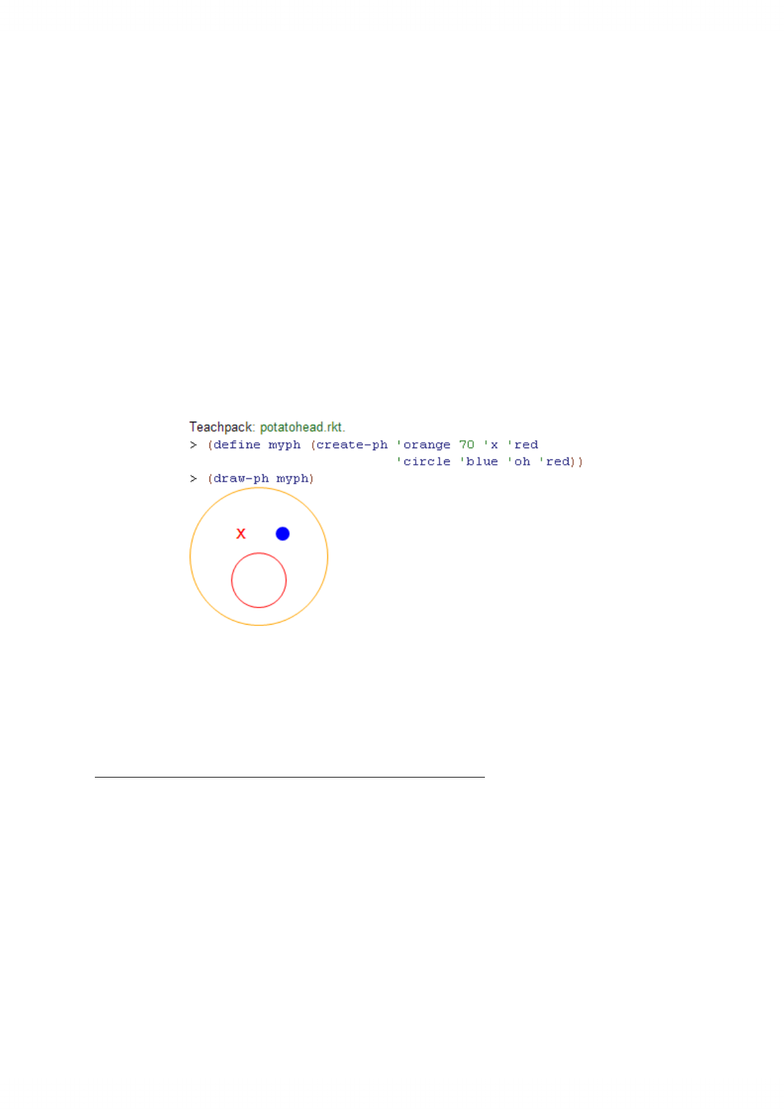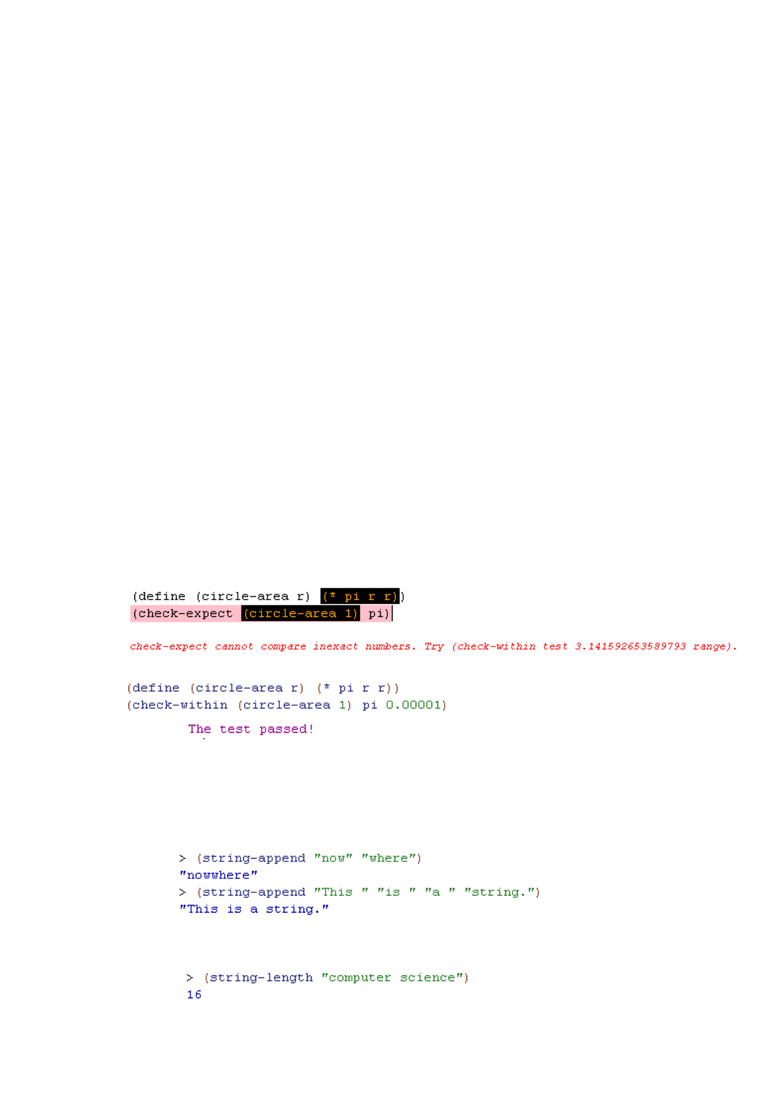Study Guides (390,000)
CA (150,000)
UW (7,000)
CS (400)
CS115 (20)
Final

# Module 1 - Module 5 The notes combines the lecture notes and the useful information in the textbook. It covers module 1 through to module 5 with all definitions, operations and examples you need.

Department
Computer Science
Course Code
CS115
Professor
Sandy Graham
Study Guide
Final

This preview shows pages 1-3. to view the full 19 pages of the document.Computer Science 115
How to Design Programs – Course Notes By: Ella Chen
Module one: Introduction to CS 115
Section 2: Numbers, Expressions, Simple Programs
2.1 Numbers and Arithmetic
Every Scheme expression is in the form (operation A … B).
Examples on mathematical operations:
- (sqrt A): square root of A
-(expt A B): A^B
- (remainder A B): remainder of A/B
- (quotient A B): quotient of A/B
- (log A): natural logarithm of A
- (sin A): sine of A radians
2.2 Variables and Programs
Defining fuctions in mathematcs:
-(define (function-name variable1 variable2…) (expression))
-E.g. (define (area-of-circle r)
(* 3.14 (* r r))
Characteristics of fuctions:
-Changing names of parameters does not change what the function does.
- The same parameter name can be used in different functions.
- The order of arguments must match the order of the parameters in the definition
of the function.
Defining constants
- (define k 3): k = 3
- (define p (* k k)) after (define k 3): (* k k) gives 9 and p is bound to 9.
Using substitution
-(define (f x) (* x x)): defines function f which consumes one numerical value;
-(define (g x y) ( - x y)): defines function g which consumes two numerical values;
- (g ( f 2) ( g 3 1)): in the form of (g x y) and x = (f 2) and y = (g 3 1).
Tracing a program
- A step-by-step simplification by applying substitution rules.
-E.g. (define (term x y) (* x (sqr y)))
(term (- 5 3) (+ 1 2)) => (term 2 (+ 1 2))
=> (term 2 3)

Only pages 1-3 are available for preview. Some parts have been intentionally blurred.=> (* 2 (sqr 3))
=> (* 2 9) => 18
The data type image (you need the world.rkt teachpack)
- use data type other than numbers
- (define image1 (rectangle 35 50 ‘solid ‘red))
- (define image2 (circle 10 ‘solid ‘blue))
- (overlay image1 image2)
- defines a head with the following attributes:
- colour, size (of the outer circle)
- left eye type, left eye colour
- right eye type,right eye colour
- mouth type, mouth colour
- the function consumes both numbers (for size) and symbols (for types and
colours).
****************************************************************************************************
************************************END OF MODULE ONE************************************
Module 2: The Design Recipe
Section 2: Numbers, Expressions, Simple Programs
2.5 Design Recipe
- information important to humans but ignored by the computer.
- Compared with CODE: code is anything that is not a comment; machine-readable.
- “;; ”: starts a line that is a comment.
- “; ”: indicates that the rest of the line is comment.
Three Comment Components of Design Recipe
- Contract: a formal description of what type of arguments the function consumes
and what type of value it produces.
;; function-name: type1 type2 type3 … typek -> type-produced

Only pages 1-3 are available for preview. Some parts have been intentionally blurred.(inputs) (output)
- Types in contracts:
- Scheme data types: num (any numeric value), image;
- User-defined data types: e.g. ph (for potatohead)
- int: integers
- nat: natural numbers (including 0)
- string: the function consumes a string
- posn: the function consumes a structure
- any: for any Scheme value
- to further specify restrictions:
- use “[ ]”, e.g. int[>=6]: integers that are greater than 6
string[len>=1]: a string of length at least 1
int[>=0,<=5]: integers from 0 to 5
- Purpose: a description of what the program consumes, what the the parameters
represent and what the porgram produces.
- Examples: illustrates the use of the program;
(function-name: argument) => produced
Test of a Function
- Use (check-expect (function-name argument) expected-output) and hit Run.
- Consider all possible cases. It’s about the quality and coverage of tests instead of
quantity.
- Check-expect only work with exact numbers. Hence the following code will result
in an ERROR:
- To avoid errors caused by inexact values, use check-within:
The Data Type String
- A string is a value made up of letters, numbers, blanks, and puctuation marks, all
enclosed in quotation marks.
- String fuctions:
- string-append: combines strings into one single string
- string-length: measures the length of a string (counts the number of
characters in the string consumed) and produces a number